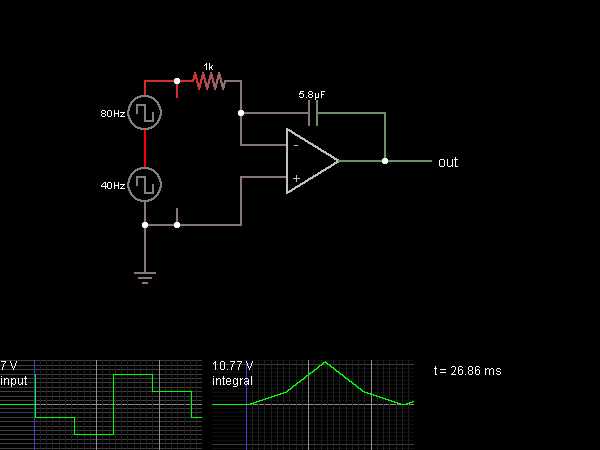Circuit Simulator - Integrator (inverting)

Where can I get Integrator (inverting) Circuit Diagram with Explanation?

IndiaBIX provides you lots of fully solved Integrator (inverting) circuit diagram with detailed explanation and working principles.

How to design a Integrator (inverting) (electronic circuit)?

You can easily design the Integrator (inverting) circuit by practicing the exercises given below. Here you can design and simulate your own electronic circuits with this Online Circuit Designer and Simulator.Circuit Description:

This circuit calculates the integral of the input, meaning that the output voltage changes at a rate proportional to the input voltage (but in the opposite direction). The op-amp attempts to keep its – input at the same voltage as the + input (which is at ground). This requires it to drain an amount of current proportional to the input voltage (V / 1000 ohms). This current goes through the capacitor, whose voltage is proportional to the integral of the current flow through it. -- Credits: Mr. Paul Falstad.